• 各个常见算法的时间复杂度
2021-07-24 21:03:09

哈希表的插入时间复杂度平均O(1),最好O(1),最差O(n)。
排序链表的插入时间复杂度平均O(n),最好O(1),最差O(n)。
数组的查询时间复杂度平均O(n),最好O(1),最差O(n)。
二叉树的查询时间复杂度平均O(logn),最好O(logn),最差O(n)。
二叉查找树的时间复杂度平均O(logn),最好O(logn),最差O(logn)。
二叉平衡树的时间复杂度平均O(logn),最好O(logn),最差O(logn)。
红黑树的时间复杂度平均O(logn),最好O(logn),最差O(logn)。
快排的时间复杂度平均O(nlogn),最好O(nlogn),最差O(n2)。
冒泡排序的时间复杂度平均O(n2),最好O(n),最差O(n2)。
插入排序的时间复杂度平均O(n2),最好O(n),最差O(n2)。
选择排序的时间复杂度平均O(n2),最好O(n2),最差O(n2)。
归并排序的时间复杂度平均O(nlogn),最好O(nlogn),最差O(n*logn)。
希尔排序的时间复杂度最好O(n),最差O(n2)。

更多相关内容
• 算法复杂度分为时间复杂度和空间复杂度。 其作用： 时间复杂度是指执行算法所需要的计算工作量； 而空间复杂度是指执行这个算法所需要的内存空间。 （算法的复杂性体现在运行该算法时的计算机所需资源的多少上，...
• 算法分析神器—时间复杂度 一套图 搞懂“时间复杂度” 目录 一、代码消耗的的时间单位分析 二、什么是时间复杂度？ 三、计算时间复杂度？ 一、得出运行时间的函数  二、对函数进行简化  四、时间复杂度排行 五、...
• 时间复杂度是算法运算所消耗的时间，因为不同大小的输入数据，算法处理所要消耗的时间是不同的，因此评估一个运行时间是比较困难的，所以通常关注的是时间频度，即算法运行计算操作的次数，记为T(n)，其中n称为...
• 时间复杂度等于m的被执行的次数: i=1时,m被执行一次： i=2时,m被执行1+3=4次： i=3时, m被执行1+3+6=10次 ： 可以观察到，第i次循环次数是一个有规律的数列: 1, 3, 6, 10,... ...  设数列 知道了第n次循环m的...
• 一、主定理 1、 主要是计算 n_log_b_a 。...复杂度是最大的。 3、n的n次方乘以log n。 Fib数列递推公式的证明（斐波那契数列） 斐波那契数列（Fibonacci sequence），又称黄金分割数列、因数学家列昂纳多·斐
• 根号n段归并排序算法时间复杂度分析过程： 1.合并 根号n向下取整 段子数组使用的是自底向上两两归并的策略 2.根号n段归并排序算法时间复杂度的数学推导
• 应用马尔科夫链模型证明了遗传禁忌搜索算法是以概率1收敛到全局最优解的,并应用求解随机算法时间复杂度的方法,即求解算法的期望收敛时间,估算了该算法的时间复杂度,结果证明该算法的时间复杂度与所得解的多样性、...
• 主要介绍了科学知识：时间复杂度计算方法,本文介绍了问题的定义、时间复杂度计算步骤、时间复杂度计算规则等内容,需要的朋友可以参考下
• 1，什么是时间复杂度？ 一个问题的规模是n，解决这一问题所需算法所需要的时间是n的一个函数T(n)，则T(n)称为这一算法的时间复杂度 2，关于时间复杂度时间复杂度是一个函数，它定性描述了该算法的运行时间。这是...
• 文章目录1 算法效率的度量方法2 函数的渐进增长3 算法时间复杂度3.1 推导大O阶方法（如何计算时间复杂度）3.2 常数阶3.2 线性阶3.3 对数阶3.4 平方阶4 常见的时间复杂度5 最坏情况和平均情况6 算法空间复杂度 ...

# 1 算法效率的度量方法

事前分析估算方法：在计算机程序编制前，依据统计方法对算法进行估算。

一个程序在计算机上运行时所消耗的时间取决于下列因素：

• 算法采用的策略、方法

• 编译产生的代码质量

• 问题的输入规模

• 机器执行指令的速度

根据以上因素，抛开与计算机硬件、软件有关的因素，一个程序的运行时间，依赖于算法的好坏和问题的输入规模。所谓问题输入规模是指输入量的多少。

// 执行了1+(n+1)+n+1=2n+3次
int i, sum = 0, n = 100; // 执行1次
for (i = 1; i <= n; i++) { // 执行了n+1次
sum = sum + i; // 执行了n次
}
printf("%d", sum); // 执行1次

// 执行了1+1+1=3次
int sum = 0, n = 100; // 执行1次
sum = (1 + n) * n/2; // 执行1次
printf("%d", sum); // 执行1次

int i, j, x = 0, sum = 0, n = 100; // 执行1次
for (i = 1; i <= n; i++) { // 内循环每一次都执行n次，两个循环执行n x n次
for (j = 1; j <= n; j++) {
x++;
sum = sum + x;
}
}
printf("%d", sum); // 执行1次


上面的程序，同样的输入规模n，求和算法的第一种，求1+2+…+n需要一段代码运行n次。那么这个问题的输入规模使得操作数量是 f(n)=n，显然运行100次的同一段代码规模是运算10次的10倍；而第二种，无论n为多少，运行次数都为1，即 f(n)=1；第三种，运算100次是运算10次的100倍，因为它是 f(n)=n²

# 2 函数的渐进增长

假设有两个算法，算法A要做2n+3次操作（可以认为是有两次n循环，然后三次赋值或打印代码），算法B要做3n+1次操作，哪种算法更快。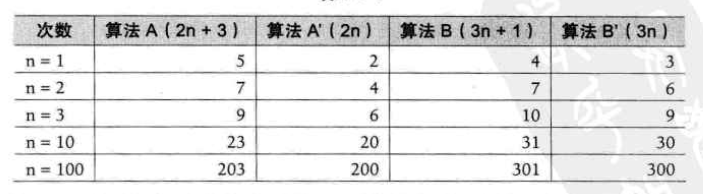根据上表的数据，输入规模n在没有限制的情况下，只要超过一个数值N，这个函数就总是大于另一个函数，我们称为函数是渐进增长的。

渐进增长：给定两个函数f(n)和g(n)，如果存在一个整数N，使得对于所有的n>N，f(n)总是比g(n)大，那么，我们说f(n)的增长渐进快于g(n)

随着n的增大，后面的算法A是+3还是算法B是+1已经不影响最终的算法变化，所以我们可以忽略这些加法常数。看最终的算法A′和算法B′就可以得出哪种算法优劣。

算法C是4n+8，算法D是2n²+1，哪个算法更快。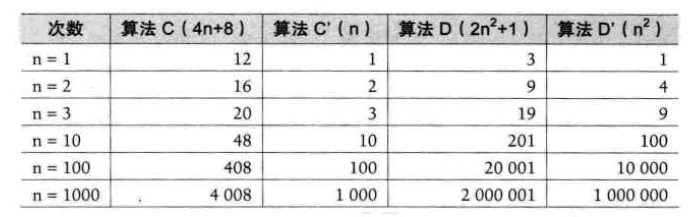根据上表的数据，随着n的增大，算法C的优势越来越优于算法D了，即使我们去掉常数发现其实结果没有发生改变，即算法C变成4n，算法D变成2n²，结果还是算法C优于算法D；甚至我们去掉与n相乘的常数，结果也没有发生改变，即最终的算法C′和算法D′展示的结果。也就是说，与最高次项相乘的常数并不重要

算法E是2n²+3n+1，算法F是2n³+3n+1，哪个算法更快。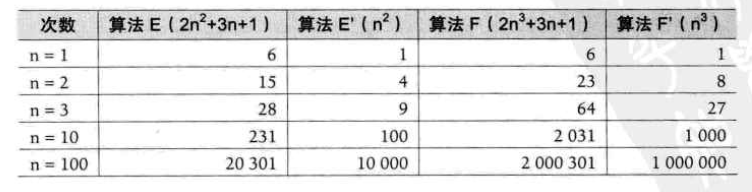根据上表的数据，可以发现，最高次项的指数大的，函数随着n的增长，结果也会增长特别快

算法G是2n²，算法H是3n+1，算法I是2n²+3n+1，哪个算法更快。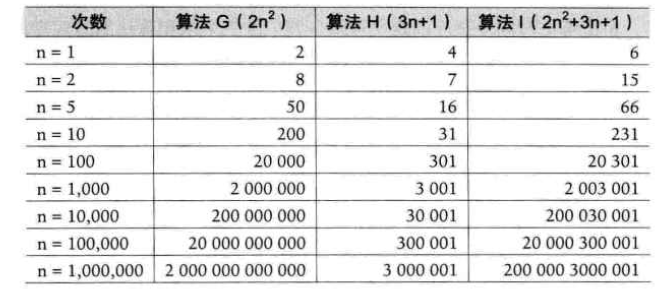根据上表的数据，当n的值越来越大时，算法H已经没法和算法G和算法I相比较了，最终几乎可以忽略不计，而算法G已经很趋近于算法I。所以可以得出结论，判断一个算法的效率时，函数中的常数和其他次要项常常可以忽略，而更应该关注主项（最高阶项）的阶数

# 3 算法时间复杂度

算法时间复杂度定义：在进行算法分析时，语句总的执行次数T(n)是关于问题规模n的函数，进而分析T(n)随n的变化情况并确定T(n)的数量级。算法的时间复杂度，也就是算法的时间度量，记作：T(n)=O(f(n))。它表示随问题规模n的增大，算法执行时间的增长率和f(n)的增长率相同，称作算法的渐近时间复杂度，简称时间复杂度。其中f(n)是问题规模n的某个函数。

用大写 O() 来体现算法时间复杂度的记法，称之为大O记法。

一般情况下，随着n的增大，T(n)增长最慢的算法为最优算法。

## 3.1 推导大O阶方法（如何计算时间复杂度）

推导大O阶可以通过以下三个步骤推算：

• 用常数1取代运行时间中的所有加法常数

• 在修改后的运行次数函数中，只保留最高阶项

• 如果最高阶项存在且不是1，则去除与这个项相乘的常数

得到的结果就是大O阶。

比如使用上面的算法G是2n²，算法H是3n+1，算法I是2n²+3n+1，经过第一二步骤后，算法G为2n²，算法H是3n，算法I是2n²，经过第三个步骤后，算法G为n²，算法H为n，算法I为n²，所以就推导出：算法G和算法I的时间复杂度为 O(n²)，算法H的时间复杂度为 O(n)

## 3.2 常数阶

int sum = 0, n = 100; // 执行1次
sum = (1+n) * n / 2; // 执行1次
printf("%d", sum); // 执行1次


上面的算法运行次数函数是f(n)=3，根据推导大O阶的方法，发现它根本没有最高阶项，所以这个算法时间复杂度为 O(1)

无论添加执行多少次 sum=(1+n) * n / 2，无论n为多少，总是该句代码的执行次数，而与n的大小无关，执行时间恒定的短发，称之为具有O(1)时间复杂度，又叫常数阶。

## 3.2 线性阶

要确定某个算法的阶次常常需要确定某个特定语句或某个语句集的运行次数。因此，我们要分析算法的复杂度，关键就是要分析循环结构的运行情况

int i;
for (i = 0; i < n; i++) { // 执行了n次，时间复杂度为O(n)
// 时间复杂度为O(1)的程序步骤序列
}


## 3.3 对数阶

// 每次count*2后距离n更近一分，即有多少个2相乘后大于n则会退出循环
// 2x=n(x为次方）,x = log2n，所以时间复杂度为O(logn)
int count = 1;
while (count < n) {
count = count * 2;
// 时间复杂度为O(1)的程序步骤序列
}


## 3.4 平方阶

// 外循环执行n次，时间复杂度为O(n)
// 内循环每次都执行n次，时间复杂度为O(n)
// 总共执行n²次，整个算法的时间复杂度为O(n²)
int i, j;
for (i = 0; i < n; i++) {
for (j = 0; j < n; j++) {
// 时间复杂度为O(1)的程序步骤序列
}
}

// 外循环执行m次，时间复杂度为O(m)
// 内循环每次执行n次，时间复杂度为O(n)
// 总共执行了nxm次，时间复杂度为O(nxm)
for (i = 0; i < m; i++) {
for (j = 0; j < n; j++) {}
}

// i=0，内循环执行了n次;i=1，内循环执行了n-1次；i=n-1时，执行了1次
// 总执行次数为n+(n-1)+(n-2)+...+1= n²/2 + n/2
// 根据大O推导，经过第一二步骤后，算法为n²/2，第三步骤去除和最高阶相乘或相除的常数
// 所以该算法的时间复杂度为O(n²)
for (i = 0; i < n; i++) {
for (j = i; j < n; j++) {}
}


# 4 常见的时间复杂度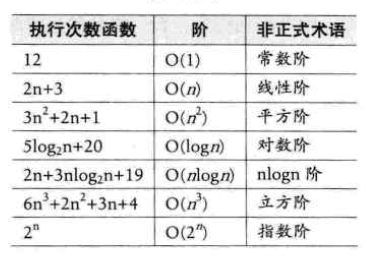常用的时间复杂度所耗费的时间从小到大依次是：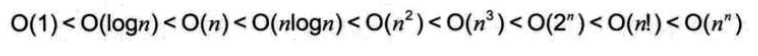在实际的项目中，一般不会去处理立方阶之后的算法也不切实际，最常见的就是常数阶、线性阶、平方阶、对数阶、nlogn阶。

# 5 最坏情况和平均情况

在算法的分析中，比如查找一个有n个随机数字数组中的某个数字，最好的情况是第一个数字就是，那么算法复杂度为O(1)，但也有可能这个数字就在最后一个位置，那么算法的时间复杂度就是O(n)。

最坏情况运行时间是一种保证，那就是运行时间将不会再坏了。在应用中，这是一种最重要的需求，通常，除非特别指定，我们提到的运行时间都是最坏情况的运行时间

而平均运行时间也就是从概率的角度看，这个数字在每一个位置的可能性是相同的，所以平均的查找时间为n/2次后发现这个目标元素。

平均运行时间是所有情况中最有意义的，因为它是期望的运行时间。在实际项目中很难通过分析得到，一般都是通过运行一定数量的实验数据后估算出来。

# 6 算法空间复杂度

算法的空间复杂度通过计算算法所需的存储空间时间，算法空间复杂度的计算公式记作：S(n)=O(f(n))，其中，n为问题的规模，f(n)为语句关于n所占存储空间的函数。

一般情况下，一个程序在机器上执行时，除了需要存储程序本身的指令、常数、变量和输入数据外，还需要存储对数据操作的存储单元。若输入数据所占空间只取决于问题本身，和算法无关，这样只需要分析该算法在实现时所需的辅助单元即可。所算法执行时所需的辅助空间相对于输入数据量而言是个常数，则称次算法为原地工作，空间复杂度为O(1)。

一般我们提到复杂度，都是说的时间复杂度，在实际的工作中，主要的还是会分析时间复杂度。

展开全文• 博客《数据结构与算法 —— 排序算法（3）》中的桶排序的时间复杂度计算公式推到过程。
• 主要给大家介绍了关于如何通过js示例讲解时间复杂度与空间复杂度的相关资料，文中通过示例代码介绍的非常详细，对大家学习或者使用js具有一定的参考学习价值，需要的朋友们下面来一起学习学习吧
• 对java的8种排序方法的空间复杂度和时间复杂度，进行了一个简单的统计java
• 算法(Algorithm)是指用来操作数据、解决程序问题的一组方法。...时间维度：是指执行当前算法所消耗的时间，我们通常用「时间复杂度」来描述。空间维度：是指执行当前算法需要占用多少内存空间，我们通常...

算法(Algorithm)是指用来操作数据、解决程序问题的一组方法。对于同一个问题，使用不同的算法，也许最终得到的结果是一样的，但在过程中消耗的资源和时间却会有很大的区别。

那么我们应该如何去衡量不同算法之间的优劣呢？

主要还是从算法所占用的「时间」和「空间」两个维度去考量。

时间维度：是指执行当前算法所消耗的时间，我们通常用「时间复杂度」来描述。空间维度：是指执行当前算法需要占用多少内存空间，我们通常用「空间复杂度」来描述。因此，评价一个算法的效率主要是看它的时间复杂度和空间复杂度情况。然而，有的时候时间和空间却又是「鱼和熊掌」，不可兼得的，那么我们就需要从中去取一个平衡点。

时间复杂度

一个算法执行所耗费的时间，从理论上是不能算出来的，必须上机运行测试才能知道。但我们不可能也没有必要对每个算法都上机测试，只需知道哪个算法花费的时间多，哪个算法花费的时间少就可以了。并且一个算法花费的时间与算法中语句的执行次数成正比例，哪个算法中语句执行次数多，它花费时间就多。一个算法中的语句执行次数称为语句频度或「时间频度」。记为T(n)。

时间频度T(n)中，n称为问题的规模，当n不断变化时，时间频度T(n)也会不断变化。但有时我们想知道它变化时呈现什么规律，为此我们引入时间复杂度的概念。算法的时间复杂度也就是算法的时间度量，记作：T(n) = O(f(n))。它表示随问题规模n的增大，算法执行时间的增长率和f(n)的增长率相同，称作算法的渐进时间复杂度，简称「时间复杂度」。

这种表示方法我们称为「 大O符号表示法」，又称为渐进符号，是用于描述函数渐进行为的数学符号

常见的时间复杂度量级有：

常数阶$O(1)$线性阶$O(n)$平方阶$O(n^2)$立方阶$O(n^3)$对数阶$O(logn)$线性对数阶$O(nlogn)$指数阶$O(2^n)$常数阶$O(1)$

$O(1)$，表示该算法的执行时间(或执行时占用空间)总是为一个常量，不论输入的数据集是大是小，只要是没有循环等复杂结构，那这个代码的时间复杂度就都是O(1)，如：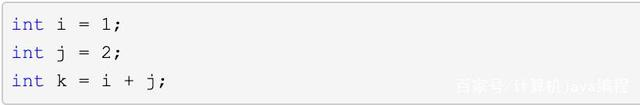上述代码在执行的时候，它消耗的时候并不随着某个变量的增长而增长，那么无论这类代码有多长，即使有几万几十万行，都可以用$O(1)$来表示它的时间复杂度。

线性阶$O(n)$

$O(n)$，表示一个算法的性能会随着输入数据的大小变化而线性变化，如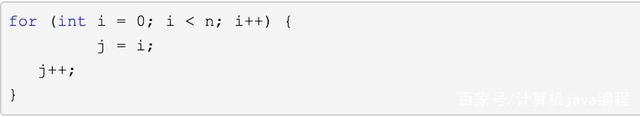这段代码，for循环里面的代码会执行n遍，因此它消耗的时间是随着n的变化而变化的，因此这类代码都可以用$O(n)$来表示它的时间复杂度。

平方阶$O(n^2)$

$O(n)$ 表示一个算法的性能将会随着输入数据的增长而呈现出二次增长。最常见的就是对输入数据进行嵌套循环。如果嵌套层级不断深入的话，算法的性能将会变为立方阶$O(n3)$，$O(n4)$，$O(n^k)$以此类推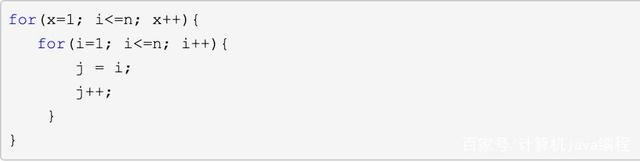指数阶$O(2^n)$

$O(2^n)$，表示一个算法的性能会随着输入数据的每次增加而增大两倍，典型的方法就是裴波那契数列的递归计算实现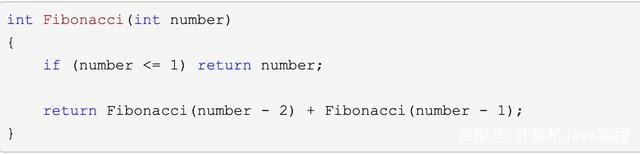对数阶$O(logn)$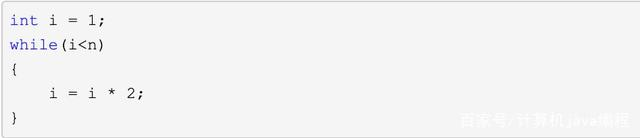上面的代码，在while循环里面，每次都将 i 乘以 2，乘完之后，i 距离 n 就越来越近了，直到i不小于n退出。我们试着求解一下，假设循环次数为x，也就是说 2 的 x 次方等于 n，则由2^x=n得出x=logn。因此这个代码的时间复杂度为$O(logn)$

线性对数阶$O(nlogn)$

线性对数阶$O(nlogn)$，就是将时间复杂度为对数阶$O(logn)$的代码循环n遍的话，那么它的时间复杂度就是 n * O(logN)，也就是了$O(nlogn)$，如下，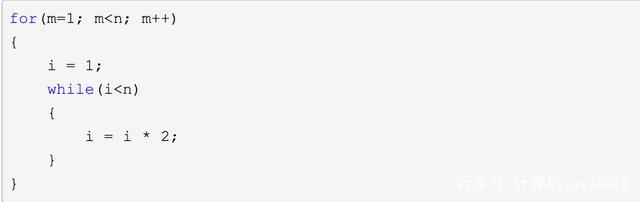除此之外，其实还有平均情况复杂度、最好时间复杂度、最坏时间复杂度。。。一般没有特殊说明的情况下，都是值最坏时间复杂度。

空间复杂度

空间复杂度(Space Complexity)是对一个算法在运行过程中临时占用存储空间大小的一个量度，同样反映的是一个趋势，一个算法所需的存储空间用f(n)表示。S(n)=O(f(n))，其中n为问题的规模，S(n)表示空间复杂度。

一个算法在计算机存储器上所占用的存储空间，包括存储算法本身所占用的存储空间，算法的输入输出数据所占用的存储空间和算法在运行过程中临时占用的存储空间这三个方面。

一般情况下，一个程序在机器上执行时，除了需要存储程序本身的指令、常数、变量和输入数据外，还需要存储对数据操作的存储单元。若输入数据所占空间只取决于问题本身，和算法无关，这样只需要分析该算法在实现时所需的辅助单元即可。若算法执行时所需的辅助空间相对于输入数据量而言是个常数，则称此算法为原地工作，空间复杂度为O(1)。当一个算法的空间复杂度与n成线性比例关系时，可表示为$0(n)$，类比时间复杂度。

空间复杂度比较常用的有：O(1)、O(n)、O(n)

空间复杂度 $O(1)$

如果算法执行所需要的临时空间不随着某个变量n的大小而变化，即此算法空间复杂度为一个常量，可表示为 O(1)举例：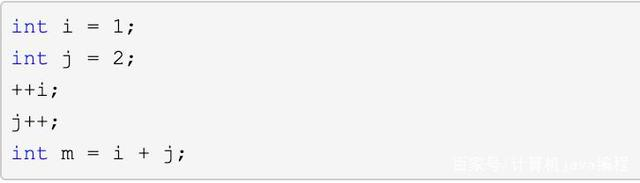代码中的 i、j、m 所分配的空间都不随着处理数据量变化，因此它的空间复杂度 S(n) = O(1)

空间复杂度 $O(n)$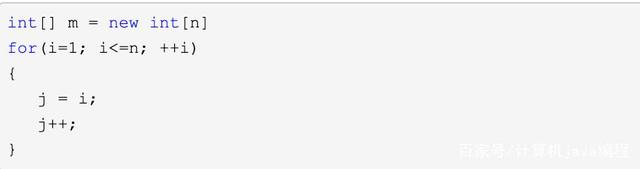这段代码中，第一行new了一个数组出来，这个数据占用的大小为n，这段代码的2-6行，虽然有循环，但没有再分配新的空间，因此，这段代码的空间复杂度主要看第一行即可，即 S(n) = O(n)

复杂度速查表

图例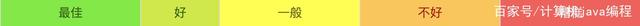大-O 复杂度曲线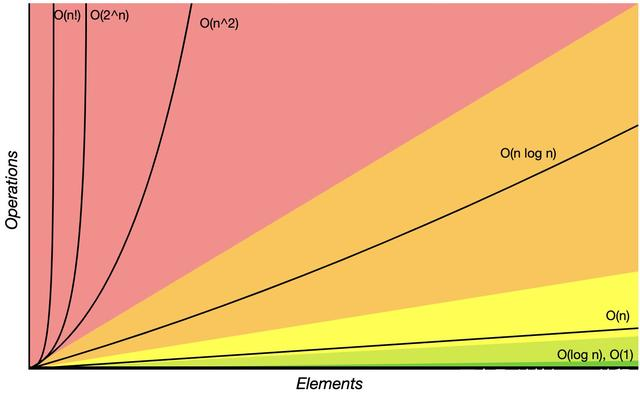抽象数据结构的操作复杂度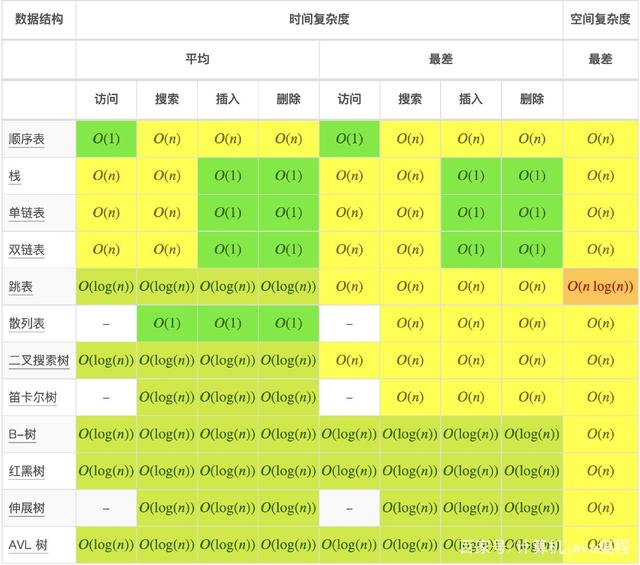数组排序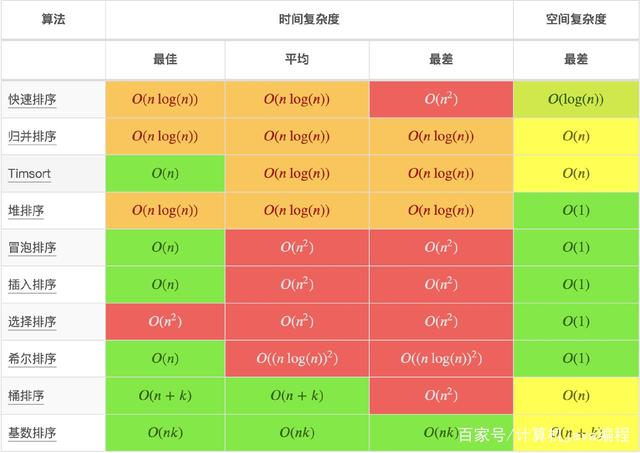图操作堆操作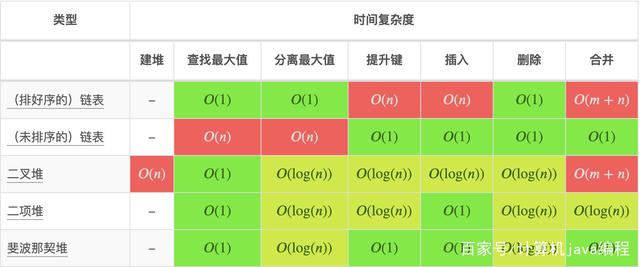展开全文• 对算法分析与设计课程的实验报告，对算法里面的时间复杂度，和增长率有很好的研究。
• NOIP普及组 提高组 CSP-J CSP-S初赛 算法的时间复杂度部分题目.pdf
• 时间复杂度与空间复杂度该怎么？这里一站式搞定！

# 时间复杂度

## 为什么要有时间复杂度？

第一、在我们的测试环境里面，不同的硬件跑出来的结果是不一样的，比如，i7与i3的机器跑同样的代码花的时间就不一样。

第二、用不同的数据去测试，那么花的时间也是不一样的。

## 通用公式

所以我们需要一套公式去计算复杂度，通用公式：T = O(N)，T表示代码执行的时间，N表示每行代码执行的次数总和，O表示所有代码的执行时间T与每行代码的执行次数总和成正比。（假设每行代码执行的时间是一样的）

O表示代码执行时间随数据规模增长的变化趋势，所以，也叫作渐进时间复杂度（asymptotic time complexity），简称时间复杂度。

### 方法一. 只关注循环执行次数最多的一段代码

int cal(int n) {
int sum = 0;
int i = 1;
for (; i <= n; ++i) {
sum = sum + i;
}
return sum;
}

其中2、3行是常量级别的代码与n的大小无关，所有不用管，执行次数最多的事第4、5行代码，所以我们只管这个两行，时间复杂度是O(n).

### 方法二、乘法法则，嵌套代码的复杂度等于内外嵌套复杂度的乘积

int cal(int n) {
int ret = 0;
int i = 1;
for (; i < n; ++i) { //第一段 这个函数的复杂度是O(n)
ret = ret + f(i);
}
}
int f(int n) {
int sum = 0;
int i = 1;
for (; i < n; ++i) {//第段段 这函数的复杂度是O(n),合起来就是O(n^2)
sum = sum + i;
}
return sum;
}

这里单看第一段函数的复杂度是O(n)，单看第二段函数的复杂度也是O(n)，有因为嵌套了，合在一起就是O(n^2)了。

### 方法三、计算复杂度是总复杂度最多的那段

int cal(int n) {
int sum_1 = 0;
int p = 1;
for (; p < 100; ++p) {//第一段为O(1)
sum_1 = sum_1 + p;
}
int sum_2 = 0;
int q = 1;
for (; q < n; ++q) {//第二段为O(n)
sum_2 = sum_2 + q;
}
int sum_3 = 0;
int i = 1;
int j = 1;
for (; i <= n; ++i) {//第三段为O(n^2)
j = 1;
for (; j <= n; ++j) { //这里又套了一层循环
sum_3 = sum_3 +  i * j;
}
}
return sum_1 + sum_2 + sum_3;
}

第一段的执行的次数是常量，所以是O(1),第二段执行了n次所以是O(n)，第三段执行了O(n2)，所以这段代码最终的时间复杂度为O(n^2)

## 再看看常见的多项式时间复杂度

### 第一种：O(1)

int i = 8;
int j = 6;
int sum = i + j;

这种情况，主要没有递归、循环，即使有上千万行的代码，时间复杂度也是O(1)

### 第二种： O(logn)、O(nlogn)

 i=1;
while (i <= n)  {
i = i * 2;
}

由上可知道，它的代码执行轨迹就是：2^1 * 2^2 * 2^3 * 2^4 …… 2^x = n 为止，那么求解这个X，就是以2为底的log函数：x=log2n，所以这段代码的时间复杂度是O(log2n)，如果在外面再套一层循环m，那么时间复杂度就是O(m*log2n)

### 第三种：O(m+n)、O(m*n)


int cal(int m, int n) {
int sum_1 = 0;
int i = 1;
for (; i < m; ++i) {//第一段复杂度为O(n)
sum_1 = sum_1 + i;
}

int sum_2 = 0;
int j = 1;
for (; j < n; ++j) {//第二段复杂度为O(m)
sum_2 = sum_2 + j;
}

return sum_1 + sum_2;
}

第一段和第二段的复杂分别是O(n)和O(m),属于同一级别，都是n级别(大于1，小于N的平方)，所以cal()方法的复杂度是O(m+n)，如果第一段和第二段有嵌套的话，那么可以相乘：O(m*n)

# 空间复杂度分析

空间复杂度就很简单了，就是一句“占用有多少个变量”就可以解释完毕！


void print(int n) {
int i = 0;
int[] a = new int[n]; // 只有这里占用了n个空间所以空间复杂度是O(n)
for (i; i <n; ++i) {
a[i] = i * i;
}

}

从上面可以看出，只有第3行 new的时候占用了n个空间，其它行占用的空间是O(1)，所以答案是O(n)

我们平时能用的空间复杂度一般是是O(1),O(n),O(n^2)，其他的O(logn)、O(nlogn)都是用不到的

# 继续拓展

=========分割线==========

复杂的时间复杂度该怎么算？有下面的问题：


// n表示数组array的长度
int find(int[] array, int n, int x) {
int i = 0;
int pos = -1;
for (; i < n; ++i) {
if (array[i] == x) {
pos = i;
break;
}
}
return pos;
}

这个空间复杂度用上面的方法显示不不行的。

如果数组中第一个元素正好是要查找的变量 x，那就不需要继续遍历剩下的 n-1 个数据了，那时间复杂度就是 O(1)。但如果数组中不存在变量 x，那我们就需要把整个数组都遍历一遍，时间复杂度就成了 O(n)。所以，不同的情况下，这段代码的时间复杂度是不一样的。

这个需要用到概率论来解决，想继续钻研的朋友可以扫码下面的二维码，听听专业老师的讲解。

展开全文数据结构 c语言 java 算法
• 本篇文章是对线性时间复杂度求数组中第K大数的方法进行了详细的分析介绍，需要的朋友参考下
• 一、什么是时间复杂度 二、时间复杂度的计算 单个循环体的推导法则 多重循环体的推导法则 多个时间复杂度的推导法则 条件语句的推导法则 习题练习 一、基础题 二、进阶题 三、再次进阶 一、什么是时间...
• 时间复杂度的理解前言定义（1）时间频度（2）时间复杂度（3）最坏时间复杂度和平均时间复杂度最坏时间复杂度和平均时间复杂度（1）最坏时间复杂度（2）平均时间复杂度尾：求时间复杂度综上： 前言 算法复杂度分为...
• 评估算法的时间复杂度的技巧小结 这篇文章献给澳门理工学院一起努力的同学们，祝大家早日摆脱算法学习的苦海，找到一叶扁舟。 什么是时间复杂度 众所周知，程序运行的时间长短跟硬件和算法都有关系。当人们想要专注...
• 本篇文章是对php中的常用算法以及时间复杂度进行了详细的分析介绍，需要的朋友参考下
• 12种排序及时间复杂度稳定性： 计数排序 基数排序 冒泡 插入 折半插入 归并 锦标赛 快速 希尔 桶排序 选择排序 堆排序排序
• ## 时间复杂度怎么算

千次阅读 2019-11-28 00:05:29
一、什么是时间复杂度？ 一个语句的频度是指该语句在算法中重复执行的次数，算法中所有语句的频度之和是关于问题规模n的函数T(n)，时间复杂度就是分析T(n)的数量级来得到的。算法的执行时间与T(n)的数量级成正比，...
• 算法的时间复杂度到底怎么? 引言 假设计算机运行一行简单语句算作一次运算。 def func1(num): print("Hello, World!\n") # 需要执行 1 次 return 0 # 需要执行 1 次 那么上面这个方法需要执行 2 次运算 def ...python 算法
• 快：时间复杂度 省：空间复杂度 1. 时间复杂度分析 1.1 实例1 def get_sum(n): result = 0 for i in range(1,n+1): result += i return result 假设每行代码对应的cpu执行时间一样，为一个时间单位unit_time ...
• 数据结构 实验报告 专业 13 物联网 年级 大二 姓名 缪思婷 学号 07 指导老师 黄河 实验室 使用日期 实验三 算法的时间复杂度 一实验目的 1帮助读者复习 C 语言程序设计中的知识 2通过本实验加深对时间复杂度的理解 ......